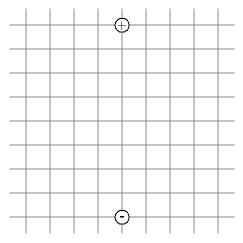### Electric Field and Potentials Pre-lab Assignment

Print this page, record your answers on it, and show it to your lab TF at the start of your lab session. In the lab you will plot field lines and equipotentials for different electrode configurations. We'll do something similar here, focusing on a dipole (a + charge and a - charge).

Click the button above to run the first simulation. You will see a fixed + charge, a fixed - charge, and four test charges that can be moved along half-circles. For each test charge, find the point on its half-circle that gives the voltage reading of the largest magnitude. Record the coordinates of that point, and the voltage reading, in the table below. The voltage reading represents the potential difference between the point where the test charge is and the center of the half-circle (i.e., the point at the center of the circle the half-circle is part of).

Electric field points in the direction of the greatest change in potential, so finding that special point on the half-circle tells you where the field at the center of the half-circle points: along the line that connects the center of the circle to the point that maximizes the potential difference.

Point # X-coordinate Y-coordinate Potential difference
1 ___________ ______________________
2 ___________ ______________________
3 ___________ ______________________
4 ___________ ______________________Double-clicking with your mouse at any point in simulation 1 will plot the field line that goes through that point. Do that at a few points to (a) check your answers to the above and (b) construct a field-line diagram that you should re-create on the diagram beside the table above.

Click the button above to run the second simulation. You will see the two fixed charges, four black test charges measuring the potential at different points, and a dragable red test charge (t) that can be moved around in the simulation using your mouse. For black test charge 1, use the movable red charge to locate several more points that have the same (or almost the same) potential reading as the test charge. Plot these points on the diagram above, and connect the points. The connected line represents an equipotential. Repeat the process for black test charges 2, 3, and 4.